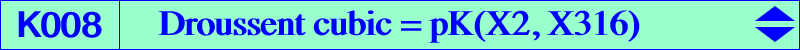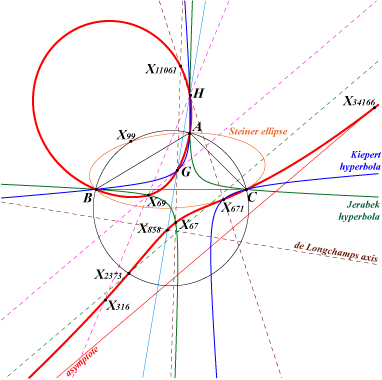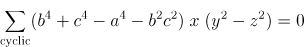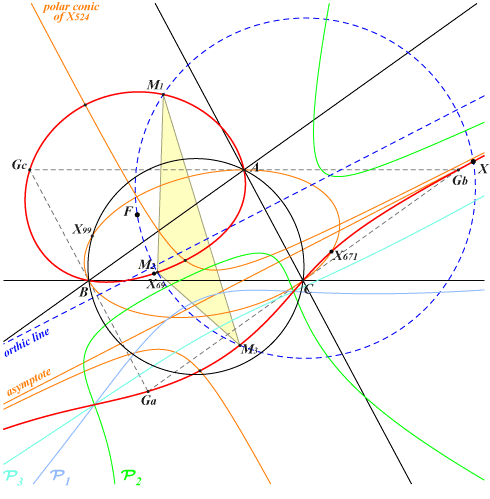X(2), X(4), X(67), X(69), X(316), X(524), X(671), X(858), X(2373), X(11061), X(13574), X(14360), X(14364), X(34163), X(34164), X(34165), X(34166), X(39157) X(55838) → X(55855), X(56471) → X(56494) Ga, Gb, Gc vertices of the antimedial triangle Other points belowThe Droussent cubic is basically the only isotomic circular pK. Its pivot is X(316) reflection of X(99) in the de Longchamps axis and isotomic conjugate of X(67). Its singular focus F = X(10748) is the midpoint of X(4)X(14360) and the intersection of the lines X(3)X(126), X(5)X(111), X(30)X(1296). K008 meets : – its real asymptote (the line X(111)X(524)) at X = X(34166) on the line X(4)X(14360). – the circumcircle at A, B, C, X(2373) and the circular points at infinity. – the Steiner ellipse at A, B, C, X(671) and two imaginary points on the de Longchamps axis (which contains X(858) as well). K008 is well-documented in Droussent's original paper. See the bibliography. See also Droussent central cubic, Droussent medial cubic and K007, property 8. The isogonal transform of the Droussent cubic is K108 = pK(X32, X23) and its antigonal transform is K273. K008 is a pivotal cubic under several conjugations : – obviously isotomic conjugation with pivot X(316), – X(316)-Ceva conjugation with pivot X(67), – X(67)-cross conjugation with pivot X(55839), – X(897)-anticomplementary conjugation with pivot X(2). Locus properties : Locus of point M whose cevian triangle is orthologic to the pedal triangle of X(23). More generally, any pK(X2,P) can be seen similarly with the pedal triangle of the isogonal of the isotomic conjugate Q of P. With Q = X(1), X(3), X(4), X(40), X(84) we obtain K034, the Lucas cubic K007, K045, K154, K133 respectively. Locus of pivots of circular pKs which pass through G and X(524). See CL035. Locus of P such that the trilinear polar of P with respect to the antimedial triangle is parallel to the polar of P in the conic with center X(67) which passes through Ga, Gb, Gc (Droussent). Locus of P such that the circles PAGa, PBGb, PCGc are concurrent (Floor van Lamoen). More generally, for any Q which is not an in/excenter, let A'B'C' be the anticevian triangle of Q.The three circles PAA', PBB', PCC' are concurrent if and only if P lies on the circular pK with pole Q^2 (or fixed point Q) and pivot according to Special Isocubics §4.2.1. For example, with Q = K, A'B'C' is the tangential triangle and the cubic is K108. Locus of P such that P, X(69) and the DF-pole of P are collinear. See CL039. See also CL051. Locus of pivots of circular pKs whose orthic line passes through X(524). The locus of the poles is K043. See CL035. See also Walsmith triangle at K1091.K008 has always three real prehessians P1, P2, P3. The centers of the polar conics of X(524) with respect to these prehessians are M1, M2, M3 where M1 = A X(671) /\ Ga X(69), M2 and M3 likewise. Furthermore the polar conic of X(524) in K008 is a rectangular hyperbola. It follows that K008 is the isogonal pK with pivot X(524) with respect to the triangle M1M2M3. Thus it must pass through the in/excenters of this latter triangle with tangents parallel to the real asymptote. X is then the isogonal conjugate of X(524) in M1M2M3 and the singular focus F is its antipode on the circumcircle of M1M2M3.This can easily be generalized for any point M on K008 as far as M is not a flex. If M1, M2, M3 are the centers of the polar conics of M with respect to the prehessians then K008 is a pivotal cubic with pivot M in the triangle M1M2M3. The tangents at M1, M2, M3 (and M) concur at the isopivot M' and the polar conic of M' contains these five points. Examples : • when M = X2, M1M2M3 is the antimedial triangle and M' is X(316). • when M = X(67), M1M2M3 is the cevian triangle of X(316) and M' is the tangential X(55839) of X(67).Points on K008 tP, X316/P, X67©P, X897-acP, tanP are respectively the isotomic conjugate, X(316)-Ceva conjugate, X(67)-cross conjugate, X(897)-anticomplementary conjugate, tangential of P.P tP X316/P X67©P X897-acP tanP SEARCH(P) 2 2 11061 14364 316 316 2.629368792488718 4 69 34163 13574 858 55847 -5.676619410926536 67 316 55839 67 11061 55839 5.987634908298794 69 4 14360 56473 524 39157 5.902289387334815 316 67 316 56730 2 67 11.5698738526503 524 671 858 56474 69 34166 5.464204286365709 671 524 55838 2373 14360 55846 1.820008131329324 858 2373 524 56476 4 56480 5.470836447670901 2373 858 55841 671 34163 13.85489574271243 11061 14364 2 56479 67 56730 -4.087163439131434 13574 14360 55844 4 55838 8.331144006674782 14360 13574 69 56478 671 55854 5.473222624215814 14364 11061 55845 2 55839 5.471194945390391 34163 56473 4 2373 3.295242497999606 34164 55851 34165 56481 34166 6.686247678169388 34165 55848 34164 56475 39157 8.137631633933725 34166 39157 55846 56480 34164 -34.48458231620383 39157 34166 55847 55854 34165 -0.6062915107828841 55838 56474 671 13574 -2.815818473943535 55839 56730 67 14364 75.35512620026191 55840 56475 55848 56480 6.355229114184416 55841 56476 2373 56473 9.906292121099521 55842 55849 55853 56491 55850 15.9845503314972 55843 56477 55850 55849 -6.54928085926222 55844 56478 13574 56474 7.185034190729121 55845 56479 14364 56730 1.886572944337355 55846 55854 34166 55851 4.626016162279342 55847 56480 39157 56494 55848 0.5422301019298273 55848 34165 55840 55851 55847 7.508058510624782 55849 55842 56485 56482 55843 5.127639031727387 55850 55855 55843 56493 55842 3.696952287299681 55851 34164 55852 55848 55846 -6.922170735786058 55852 56481 55851 55854 4.211444953165199 55853 56482 55842 55855 9.005399048654538 55854 55846 39157 55852 20.3292923875051 55855 55850 56488 56477 55853 1.189187408875814 56471 56483 56472 56472 56484 56471 56473 34163 69 55841 1.833740488379655 56474 55838 524 55844 -18.03575067156534 56475 55840 34165 56476 55841 858 56477 55843 55855 56485 56478 55844 14360 56479 55845 55840 56480 55847 34166 55840 -64.20749063836018 56481 55852 34164 56482 55853 55849 56488 56483 56471 56484 56484 56472 56483 56485 56491 56477 56486 56492 56490 56487 56490 56494 56488 56493 55855 56482 56489 56494 56490 56492 56490 56847 56491 56845 55842 56492 56846 56849 56493 56848 55850 56494 56849 56847 56730 55839 316 55845 2.784738933404024